## Helmholtz Differential Equation--Elliptic Cylindrical Coordinates

In Elliptic Cylindrical Coordinates, the Scale Factors are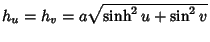,, and the separation functions are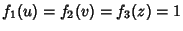, giving a Stäckel Determinant of. The Helmholtz differential equation is(1)

Attempt Separation of Variables by writing(2)

then the Helmholtz Differential Equation becomes(3)

Now divide by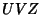to give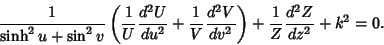(4)

Separating thepart,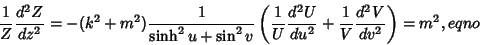(5)

so(6)

which has the solution(7)

Rewriting (5) gives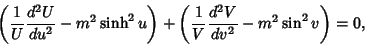(8)

which can be separated into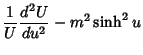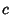(9)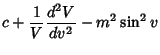(10)

so(11)(12)

Now use(13)(14)

to obtain(15)(16)

Regrouping gives(17)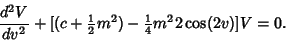(18)

Letand, then these become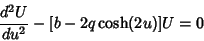(19)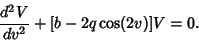(20)

Here, (20) is the Mathieu Differential Equation and (19) is the modified Mathieu Differential Equation. These solutions are known as Mathieu Functions.